# Python Turtle

Turtle is a Python library to draw different graphical shapes.Turtle library in Python to draw graphic shapes like triangles, squares , stars multiple shapes

``````import turtle
my_w=turtle.Turtle()
my_w.forward(200) # draw line of 200px distance
turtle.done()``````

## Triangle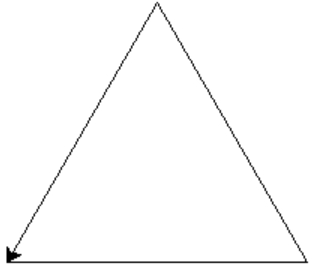``````import turtle
my_t = turtle.Turtle()

my_t.forward(200)
my_t.right(-120)
my_t.forward(200)
my_t.right(-120)
my_t.forward(200)

turtle.done()``````

## Square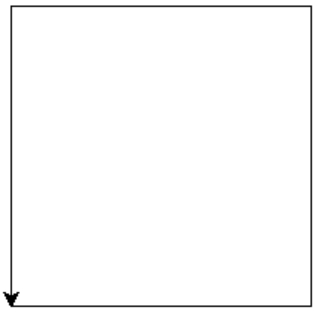``````import turtle
my_t = turtle.Turtle()

my_t.forward(200)
my_t.right(-90)
my_t.forward(200)
my_t.right(-90)
my_t.forward(200)
my_t.right(-90)
my_t.forward(200)

turtle.done()``````

## Hexagon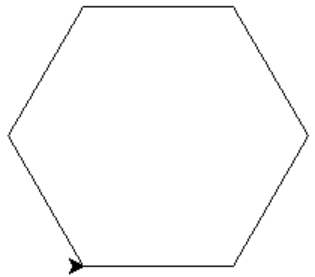``````import turtle
my_t = turtle.Turtle()
for i in range (6):
my_t.forward(100)
my_t.right(-60)

turtle.done()``````

## Drawing star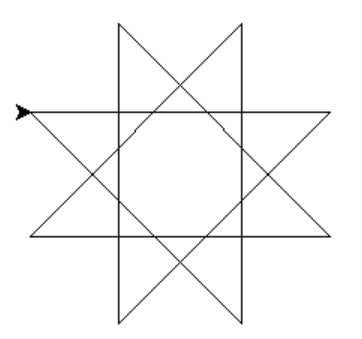``````import turtle
my_w=turtle.Turtle()

for i in range(8):
my_w.forward(200)
my_w.right(135)

turtle.done()``````

## Multiple stars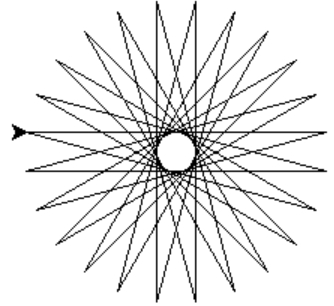``````import turtle
my_w=turtle.Turtle()

for i in range(24):
my_w.forward(200)
my_w.right(165)

turtle.done()``````

## Multiple triangles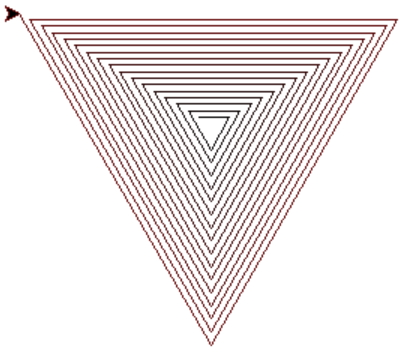``````import turtle
my_t = turtle.Turtle()
turtle.colormode(255)
#turtle.speed(9)
x=20
r,g,b=10,10,10
d=2
for i in range (48):
my_t.pencolor(r,g,b)
my_t.forward(x)
my_t.right(120)
r=r+d
x=x+5
turtle.done()``````

## Multiple Squares``````import turtle
my_t = turtle.Turtle()
turtle.colormode(255)
#turtle.speed(9)
x=20
r,g,b=10,10,10
d=2
for i in range (48):
my_t.pencolor(r,g,b)
my_t.forward(x)
my_t.right(90)
r=r+d
x=x+5
turtle.done()``````

Subscribe to our YouTube Channel here

## Subscribe

* indicates required
Subscribe to plus2netplus2net.com